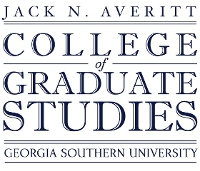## Electronic Theses and Dissertations

Spring 2010

#### Degree Name

Master of Science in Mathematics (M.S.)

#### Document Type and Release Option

Thesis (restricted to Georgia Southern)

#### Department

Department of Mathematical Sciences

Xiezhang Li

Jiehua Zhu

Yan Wu

n/a

#### Abstract

We propose and study the reconstruction of large binary images in discrete tomography (DT) by applying the binary steering scheme to the block-iterative projections methods for solving an underdetermined linear system Ax = b. Three algorithms: Algebraic Reconstruction Technique (ART) Algorithm, Cimmino's Algorithm (CIM) with Equal Weights Algorithm and Fully-Simultaneous Diagonally-Relaxed Orthogonal Projections (DROP) Algorithm are modified. We develop an efficient storage of the binary sparse system matrix A and analyze the work load of the fast execution of the matrix-vector multiplication Ax. Numerical experiments are given to illustrate faster convergence by binary steering block iterations and efficient storage of A.

No

COinS